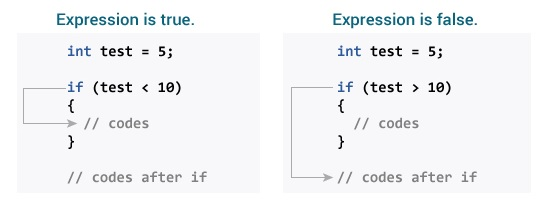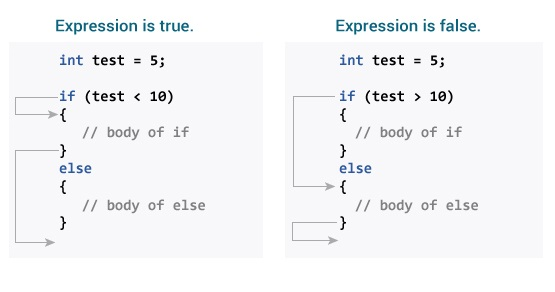In programming, it is often desirable to execute a certain piece of code based on whether the specified condition is true or false (which is specified only at runtime).

For such cases, control flow commands are used.

Command if (if-then in Java

The syntax of the if-then command in Java is as follows:

if (expression) {

// statements

}

Here expression is a boolean expression (true or false).

If the expression is evaluated correctly, the statements inside the if body (statements inside parentheses) are executed.

If the expression is evaluated incorrectly, the statements inside the if body will not be executed.

How does the if statement work?Example 1: If statement in Java

1. class IfStatement {
2. public static void main (String [] args) {
3. int number = 10;
4. if (number> 0) {
5. System.out.println (“Number is positive.”);
6. }
7. System.out.println (“This statement is always executed.”);
8. }
9. }

Output

Number is positive.

This statement is always executed.

When the number is equal to 10, the expression number> 0 is considered correct. Hence, the code inside the if body is executed.

Now change the number value to a negative integer. For example -5. The output in this case will be equal to:

This statement is always executed.

When the number is equal to -5, the expression number> 0 is considered incorrect. Therefore, the Java compiler overrides the if body commands.

Command (if… else (if-then-else in Java)

In if, if the condition is evaluated correctly, a certain part of the code will be executed. The if statement may also have an else block. If the condition is evaluated incorrectly, the commands in the else body are executed.

The syntax of the if-then-else command is as follows:

if (expression) {

// codes

}

else {

// some other code

}

How does the if… else command work?Example 2: The if… else command in Java

1. class IfElse {
2. public static void main (String [] args) {
3. int number = 10;
4. if (number> 0) {
5. System.out.println (“Number is positive.”);
6. }
7. else {
8. System.out.println (“Number is not positive.”);
9. }
10. System.out.println (“This statement is always executed.”);
11. }
12. }

Output

Number is positive.

This statement is always executed.

When number is equal to 10, the expression number> 0 is evaluated correctly. In this case, the code inside the if body will be executed and the code inside the else body will not be executed.

Now change the value of the number to a negative number. For example -5. The output in this case will be equal to:

Number is not positive.

This statement is always executed.

When the number is -5, the expression number> 0 is evaluated incorrectly. In this case, the code inside the else body will be executed and the code inside the if body will not be executed.

The command if else… if in Java

In Java, it is possible to execute one piece of code out of many codes. You can use if… else… if to do this.

if (expression1)

{

// codes

}

else if (expression2)

{

// codes

}

else if (expression3)

{

// codes

}

.

.

else

{

// codes

}

If statements are executed from top to bottom. As soon as the condition is correct, the corresponding code is executed. After that, the program control jumps out of if… else… if.

If all test statements are incorrect, the code inside the else body will be executed.

Example 3: If else… if statement in Java

2. public static void main (String [] args) {
3. int number = 0;
4. if (number> 0) {
5. System.out.println (“Number is positive.”);
6. }
7. else if (number <0) {
8. System.out.println (“Number is negative.”);
9. }
10. else {
11. System.out.println (“Number is 0.”);
12. }
13. }
14. }

Output

Number is 0.

When number is 0, both number> 0 and number <0 are considered incorrect. Hence, the code is executed inside the body of else.

The above program checks whether the number is positive, negative or 0.

The if… else command nested in Java

It is possible to have nested if..else commands in Java.

Here is a program to find the largest number out of 3:

Example 4: If دستور else nested command

1. class Number {
2. public static void main (String [] args) {
3. Double n1 = -1.0, n2 = 4.5, n3 = -5.3, largestNumber;
4. if (n1> = n2) {
5. if (n1> = n3) {
6. largestNumber = n1;
7. } else {
8. largestNumber = n3;
9. }
10. } else {
11. if (n2> = n3) {
12. largestNumber = n2;
13. } else {
14. largestNumber = n3;
15. }
16. }
17. System.out.println (“Largest number is” + largestNumber);
18. }
19. }

Output

Largest number is 4.5

Note: In the above programs, we have assigned values ​​to the variables ourselves to make this easier. However, in real-world applications, these values ​​may be derived from user input data, log files, submitted forms, and so on.